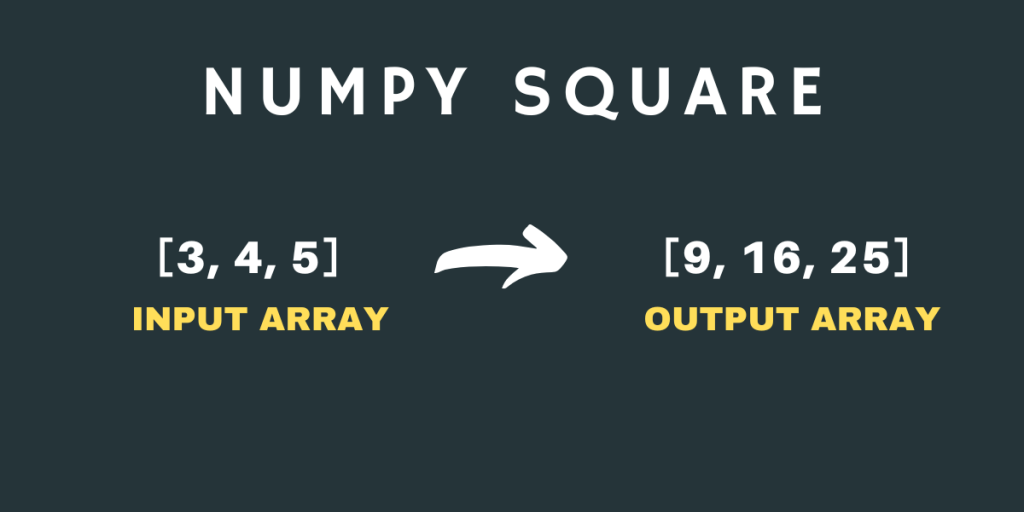# numpy.square() – Explained in a Simple WayHello everyone! In this tutorial, we will be learning about the NumPy square function. This is a simple function to understand and easy to use. So, let’s get started.

## What is NumPy square?

`NumPy square` is one of the Mathematical Functions of the NumPy Library that calculates the square value of the input number. Yes, it’s that simple by the definition. It can be used with both real and complex numbers. The square of a number is equal to the number multiplied by itself.

## Syntax of NumPy square

Let’s look at the syntax of the NumPy square function.

```numpy.square(input)
```

Here, the input can be a single number, a NumPy array of numbers as well as a complex number. Let’s write code to understand the function better.

## Working with NumPy square

Let’s now start working on the Numpy.square() method to understand it through examples.

### NumPy square of a single number

```import numpy as np

print("Square of 5 is :",np.square(-5))
print("Square of 2.5 is :",np.square(2.5))
print("Square of 10 is :",np.square(10))
print("Square of 13.345 is :",np.square(13.345))
print("Square of -19.93 is :",np.square(-19.93))
```

Output

```Square of 5 is : 25
Square of 2.5 is : 6.25
Square of 10 is : 100
Square of 13.345 is : 178.08902500000002
Square of -19.93 is : 397.2049
```

The above outputs are pretty obvious. In the last example, we have passed a negative number as input to the function and the output is a positive number.

### NumPy square of a NumPy array of numbers

Let’s look at some examples where we will use the NumPy array with the function.

```import numpy as np

# NumPy Array of Integral Values
a = np.array((3 , -4 , 5 , 12 , 499))

print("Input Array:\n",a)
print("Square Values:\n",np.square(a))

# NumPy Array of Floating Point Values
b = np.array((0.43 , -2.5 , 9.23 , 4.57 , 6.5))

print("Input Array:\n",b)
print("Square Values:\n",np.square(b))
```

Output

```Input Array:
[  3  -4   5  12 499]
Square Values:
[     9     16     25    144 249001]
Input Array:
[ 0.43 -2.5   9.23  4.57  6.5 ]
Square Values:
[ 0.1849  6.25   85.1929 20.8849 42.25  ]
```

There is one task for you all, you have to use the NumPy square function with a 2-D NumPy array and observe the output.

### NumPy square with Complex Numbers

Now, let’s use the NumPy square function with Complex Numbers.

```import numpy as np

print("The square of 4+5j is:",np.square(4+5j))

print("The square of -1+0.5j is:",np.square(-1+0.5j))
```

Output

```The square of 4+5j is: (-9+40j)
The square of -1+0.5j is: (0.75-1j)
```

The mathematics behind the calculation of the squares of the Complex Number is really interesting. It uses some basic formulae and simple identities of Complex Numbers.

## Graphical Representation of NumPy square

Now, let’s plot the NumPy square curve using Matplotlib Library.

```import numpy as np

import matplotlib.pyplot as plt

a = np.linspace(-10 , 10 , 30)

b = np.square(a)

plt.plot(a , b , color = "green" , marker = "o")
plt.title("numpy.square()")
plt.xlabel("X")
plt.ylabel("Y")
plt.show()
```

Output